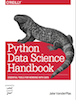This is an excerpt from the Python Data Science Handbook by Jake VanderPlas; Jupyter notebooks are available on GitHub.

The text is released under the CC-BY-NC-ND license, and code is released under the MIT license. If you find this content useful, please consider supporting the work by buying the book!

# Introduction to NumPy

This chapter, along with chapter 3, outlines techniques for effectively loading, storing, and manipulating in-memory data in Python. The topic is very broad: datasets can come from a wide range of sources and a wide range of formats, including be collections of documents, collections of images, collections of sound clips, collections of numerical measurements, or nearly anything else. Despite this apparent heterogeneity, it will help us to think of all data fundamentally as arrays of numbers.

For example, images–particularly digital images–can be thought of as simply two-dimensional arrays of numbers representing pixel brightness across the area. Sound clips can be thought of as one-dimensional arrays of intensity versus time. Text can be converted in various ways into numerical representations, perhaps binary digits representing the frequency of certain words or pairs of words. No matter what the data are, the first step in making it analyzable will be to transform them into arrays of numbers. (We will discuss some specific examples of this process later in Feature Engineering)

For this reason, efficient storage and manipulation of numerical arrays is absolutely fundamental to the process of doing data science. We'll now take a look at the specialized tools that Python has for handling such numerical arrays: the NumPy package, and the Pandas package (discussed in Chapter 3).

This chapter will cover NumPy in detail. NumPy (short for Numerical Python) provides an efficient interface to store and operate on dense data buffers. In some ways, NumPy arrays are like Python's built-in list type, but NumPy arrays provide much more efficient storage and data operations as the arrays grow larger in size. NumPy arrays form the core of nearly the entire ecosystem of data science tools in Python, so time spent learning to use NumPy effectively will be valuable no matter what aspect of data science interests you.

If you followed the advice outlined in the Preface and installed the Anaconda stack, you already have NumPy installed and ready to go. If you're more the do-it-yourself type, you can go to http://www.numpy.org/ and follow the installation instructions found there. Once you do, you can import NumPy and double-check the version:

In :
import numpy
numpy.__version__

Out:
'1.11.1'

For the pieces of the package discussed here, I'd recommend NumPy version 1.8 or later. By convention, you'll find that most people in the SciPy/PyData world will import NumPy using np as an alias:

In :
import numpy as np


Throughout this chapter, and indeed the rest of the book, you'll find that this is the way we will import and use NumPy.

## Reminder about Built In Documentation¶

As you read through this chapter, don't forget that IPython gives you the ability to quickly explore the contents of a package (by using the tab-completion feature), as well as the documentation of various functions (using the ? character – Refer back to Help and Documentation in IPython).

For example, to display all the contents of the numpy namespace, you can type this:

In : np.<TAB>


And to display NumPy's built-in documentation, you can use this:

In : np?


More detailed documentation, along with tutorials and other resources, can be found at http://www.numpy.org.